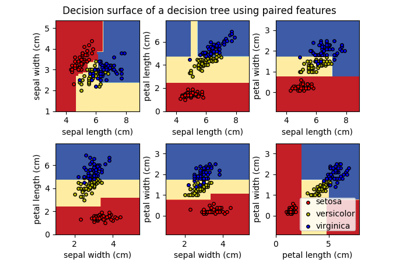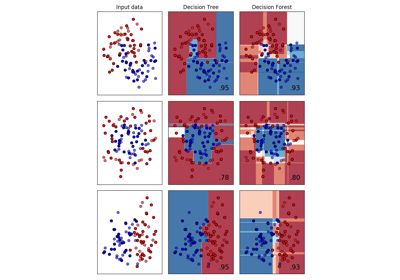# `koho.sklearn`.DecisionForestClassifier¶

class `koho.sklearn.``DecisionForestClassifier`(n_estimators=100, bootstrap=False, oob_score=False, class_balance='balanced', max_depth=3, max_features='auto', max_thresholds=None, missing_values=None, random_state=None, n_jobs=None)[source]

A decision forest classifier.

Parameters
• n_estimators (int, optional (default=100)) – The number of decision trees in the forest.

• bootstrap (boolean, optional (default=False)) – Whether bootstrap samples are used when building trees. Out-of-bag samples are used to estimate the generalization accuracy.

• oob_score (bool, optional (default=False)) – Whether to use out-of-bag samples to estimate the generalization accuracy.

• class_balance (str 'balanced' or None, optional (default='balanced')) –

Weighting of the classes.

• If ‘balanced’, then the values of y are used to automatically adjust class weights inversely proportional to class frequencies in the input data.

• If None, all classes are supposed to have weight one.

• max_depth (int or None, optional (default=3)) –

The maximum depth of the tree.

• If None, the depth of the tree is expanded until all leaves are pure or no further impurity improvement can be achieved.

• max_features (int, float, str or None, optional (default=None)) –

The number of random features to consider when looking for the best split at each node.

• If int, then consider `max_features` features.

• If float, then `max_features` is a percentage and int(`max_features` * n_features) features are considered.

• If ‘auto’, then `max_features` = sqrt(n_features).

• If ‘sqrt’, then `max_features` = sqrt(n_features).

• If ‘log2’, then `max_features` = log2(n_features).

• If None, then `max_features` = n_features considering all features in random order.

Note: the search for a split does not stop until at least one valid partition of the node samples is found up to the point that all features have been considered, even if it requires to effectively inspect more than `max_features` features.

Decision Tree: `max_features` = None and `max_thresholds` = None

Random Tree: `max_features` < n_features and `max_thresholds` = None

• max_thresholds (int 1 or None, optional (default=None)) –

The number of random thresholds to consider when looking for the best split at each node.

• If 1, then consider 1 random threshold, based on the Extreme Randomized Tree formulation.

• If None, then all thresholds, based on the mid-point of the node samples, are considered.

Extreme Randomized Trees (ET): `max_thresholds` = 1

Totally Randomized Trees: `max_features` = 1 and `max_thresholds` = 1, very similar to Perfect Random Trees (PERT).

• missing_values (str 'NMAR' or None, optional (default=None)) –

Handling of missing values, defined as np.NaN.

• If ‘NMAR’ (Not Missing At Random), then during training: the split criterion considers missing values as another category and samples with missing values are passed to either the left or the right child depending on which option provides the best split, and then during testing: if the split criterion includes missing values, a missing value is dealt with accordingly (passed to left or right child), or if the split criterion does not include missing values, a missing value at a split criterion is dealt with by combining the results from both children proportionally to the number of samples that are passed to the children during training.

• If None, an error is raised if one of the features has a missing value. An option is to use imputation (fill-in) of missing values prior to using the decision tree classifier.

• random_state (int or None, optional (default=None)) –

A random state to control the pseudo number generation and repetitiveness of fit().

• If int, random_state is the seed used by the random number generator;

• If None, the random number generator is seeded with the current system time.

• n_jobs (int, optional (default=None)) –

The number of jobs to run in parallel for both fit and predict.

• None means 1.

• If -1, then the number of jobs is set to the number of cores.

`n_outputs_`

The number of outputs (multi-output).

Type

int

`classes_`

The classes labels for each output.

Type

list of variable size arrays, shape = [n_classes for each output]

`n_classes_`

The number of classes for each output.

Type

list of int

`n_features_`

The number of features.

Type

int

`estimators_`

The collection of the underlying sub-estimators.

Type

list of tree objects from DecisionTreeClassifier

`feature_importances_`

The feature importances. The higher, the more important the feature. The importance of a feature is computed as the (normalized) total reduction of the criterion brought by that feature.

Type

array, shape = [n_features]

`oob_score_`

Score of the training dataset obtained using an out-of-bag estimate.

Type

float

`__init__`(n_estimators=100, bootstrap=False, oob_score=False, class_balance='balanced', max_depth=3, max_features='auto', max_thresholds=None, missing_values=None, random_state=None, n_jobs=None)[source]

Create a new decision forest classifier and initialize it with hyperparameters.

property `feature_importances_`

Get feature importances from the decision forest.

`fit`(X, y)[source]

Build a decision forest classifier based on decision tree classifiers from the training data.

Parameters
• X (array, shape = [n_samples, n_features]) – The training input samples.

• y (array, shape = [n_samples] or [n_samples, n_outputs]) – The target class labels corresponding to the training input samples.

Returns

self – Returns self.

Return type

object

`get_params`(deep=True)

Get parameters for this estimator.

Parameters

deep (boolean, optional) – If True, will return the parameters for this estimator and contained subobjects that are estimators.

Returns

params – Parameter names mapped to their values.

Return type

mapping of string to any

`predict`(X)[source]

Predict classes for the test data using soft voting.

Parameters

X (array, shape = [n_samples, n_features]) – The test input samples.

Returns

y – The predicted classes for the test input samples.

Return type

array, shape = [n_samples] or [n_samples, n_outputs]

`predict_proba`(X)[source]

Predict classes probabilities for the test data.

Parameters

X (array, shape = [n_samples, n_features]) – The test input samples.

Returns

p – The predicted classes probablities for the test input samples.

Return type

array, shape = [n_samples, n_classes]

`score`(X, y)[source]

Returns the mean accuracy on the given test data and labels.

sklearn has no metrics support for “multiclass-multioutput” format, therefore we implement our own score() here

In multi-label classification, this is the subset accuracy which is a harsh metric since you require for each sample that each label set be correctly predicted.

Parameters
• X (array-like, shape = (n_samples, n_features)) – Test samples.

• y (array-like, shape = (n_samples) or (n_samples, n_outputs)) – True labels for X.

Returns

score – Mean accuracy of self.predict(X) wrt. y.

Return type

float

`set_params`(**params)

Set the parameters of this estimator.

The method works on simple estimators as well as on nested objects (such as pipelines). The latter have parameters of the form `<component>__<parameter>` so that it’s possible to update each component of a nested object.

Returns

Return type

self

## Examples using `koho.sklearn.DecisionForestClassifier`¶